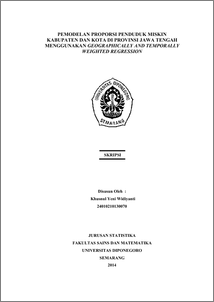# PEMODELAN PROPORSI PENDUDUK MISKIN KABUPATEN DAN KOTA DI PROVINSI JAWA TENGAH MENGGUNAKAN GEOGRAPHICALLY AND TEMPORALLY WEIGHTED REGRESSION

Widiyanti, Khusnul Yeni (2014) PEMODELAN PROPORSI PENDUDUK MISKIN KABUPATEN DAN KOTA DI PROVINSI JAWA TENGAH MENGGUNAKAN GEOGRAPHICALLY AND TEMPORALLY WEIGHTED REGRESSION. Undergraduate thesis, FSM Universitas Diponegoro.Preview
PDF
667Kb

## Abstract

Regression analysis is a statistical analysis that aims to quantify the effect of predictor variables on the response variable. Geographically Weighted Regression (GWR) is a local form of regression and a statistical method used to analyze spatial data. Geographically and Temporally Weighted Regression (GTWR) is the development of GWR models to handle data that is not stationary both in terms of spatial and temporal simultaneously. In obtaining estimates of parameters of the GTWR model can be used Weighted Least Square method (WLS). Selection of the optimum bandwidth used method of Cross Validation (CV). Conformance testing global regression and GTWR models approximated by the distribution of F, whereas the partial testing of the model parameters using the t distribution. Application GTWR models at the level of poverty in Central Java province in 2008 to 2012 showed GTWR models differ significantly from the global regression model. Based on R2 and Mean Squared Error (MSE) value between the global regression model and GTWR models, it is known that the GTWR model with exponential weighting kernel function is the best model is used to analyze the proportion of poor people in Central Java province in 2008 to 2012 because it has a value of R2 larger and MSE is the smallest. Keywords: Bandwidth, Cross Validation, Exponential Kernel Functions, Geographically and Temporally Weighted Regression, Weighted Least Square, R2, Mean Squared Error.

Item Type: Thesis (Undergraduate) H Social Sciences > HA Statistics Faculty of Science and Mathematics > Department of Statistics 46332 Mr Hasbi Yasin 09 Sep 2015 16:19 09 Sep 2015 16:19

Repository Staff Only: item control page